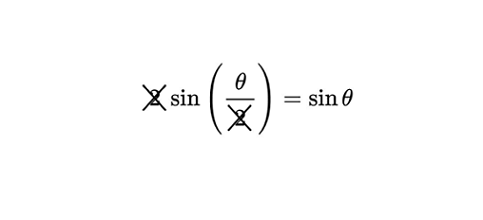# It's just 2 cut off with 2 right?

Geometry Level 2Mandy was learning trigonometric identities one day and erroneously decided that $2\sin\left(\frac\theta2\right)=\sin\theta$ was true for all $\theta$. What is the sum of all values of $\theta$ in the interval $[0^\circ,360^\circ]$ for which this equation is actually true?

×